# D Block elements Questions and Answers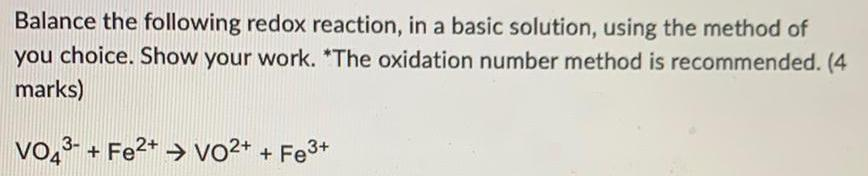Inorganic Chemistry
D Block elements
Balance the following redox reaction in a basic solution using the method of you choice Show your work The oxidation number method is recommended 4 marks VO43 Fe VO Fe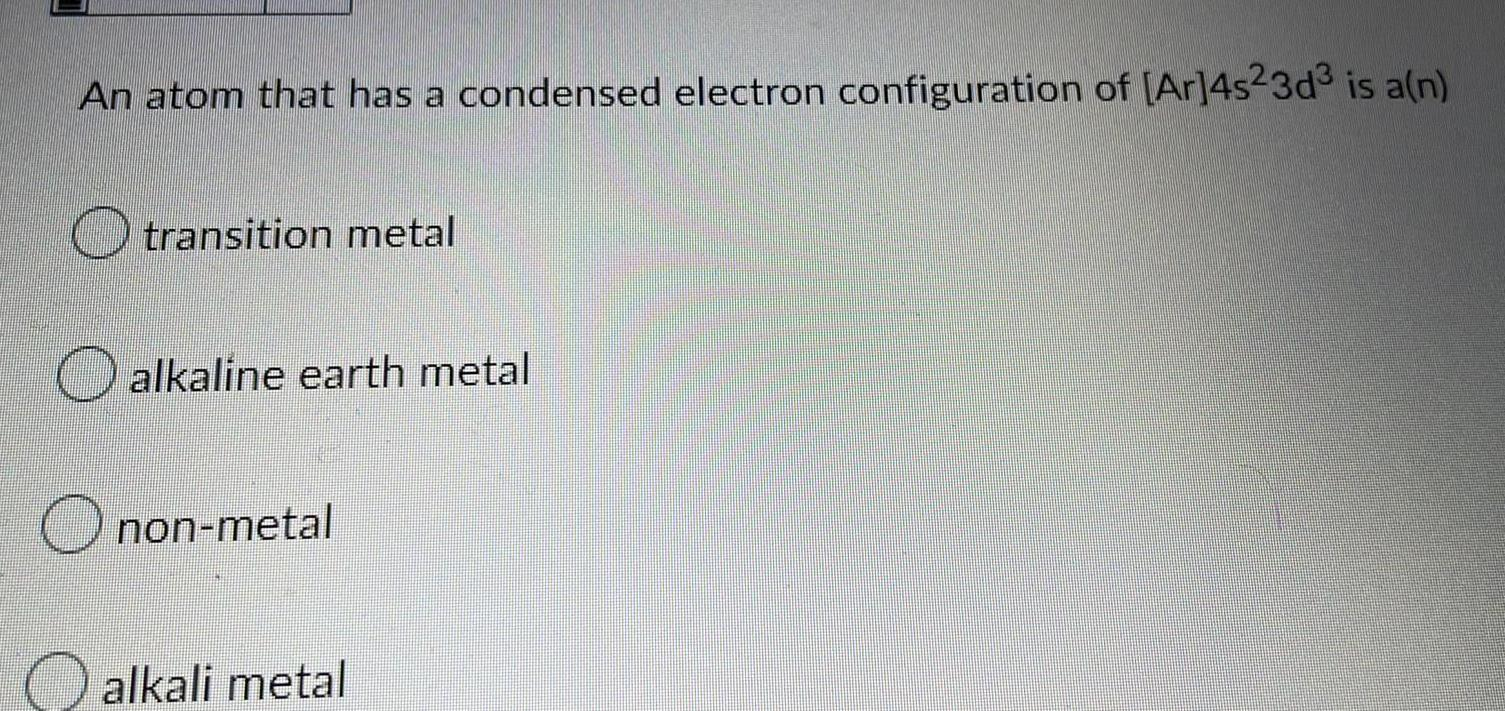Inorganic Chemistry
D Block elements
An atom that has a condensed electron configuration of Ar 4s23d is a n transition metal alkaline earth metal Onon metal alkali metal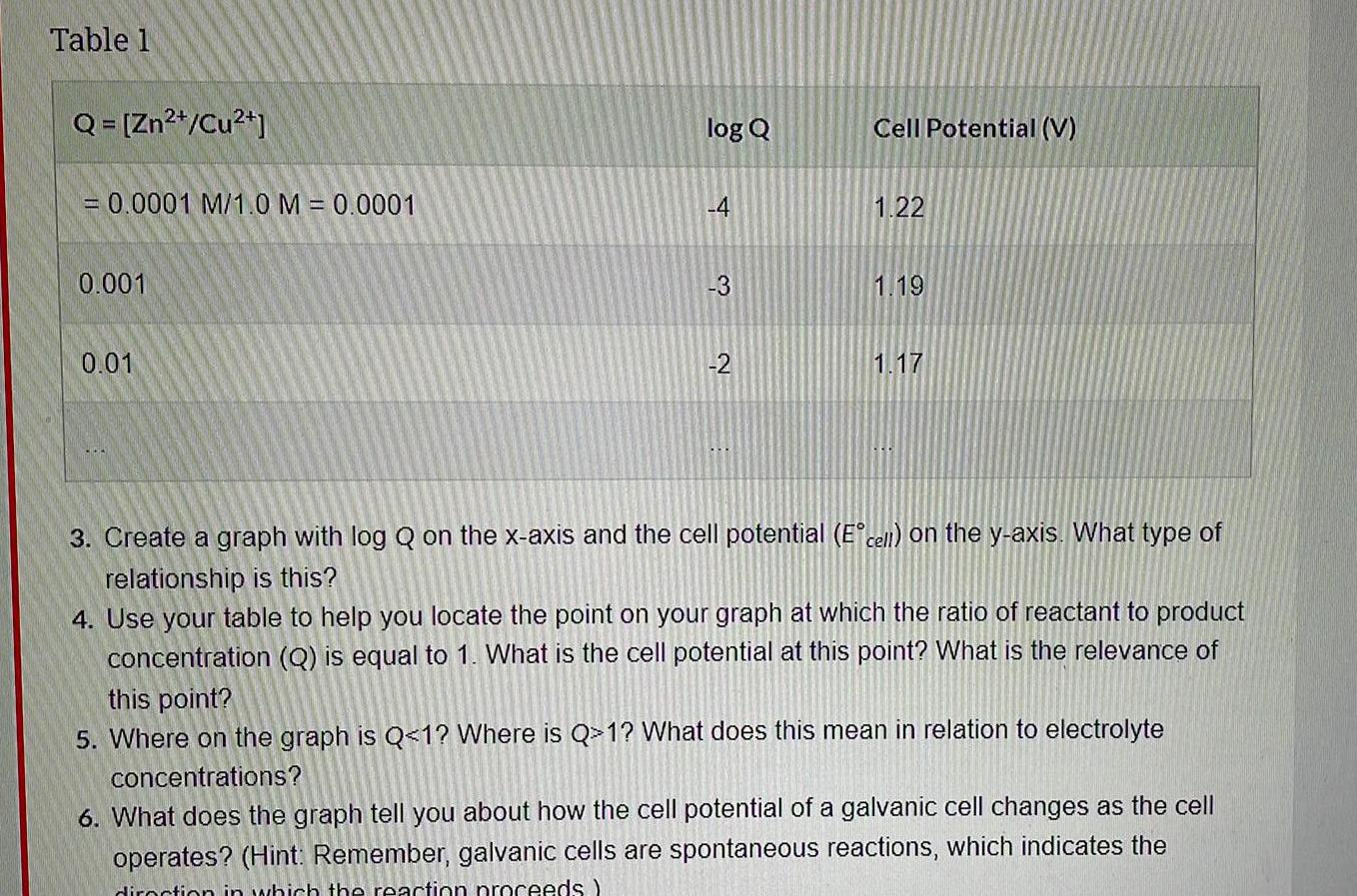Inorganic Chemistry
D Block elements
Table 1 Q Zn Cu 0 0001 M 1 0 M 0 0001 0 001 0 01 log Q 4 3 2 Cell Potential V 1 22 1 19 1 17 3 Create a graph with log Q on the x axis and the cell potential E cell on the y axis What type of relationship is this 4 Use your table to help you locate the point on your graph at which the ratio of reactant to product concentration Q is equal to 1 What is the cell potential at this point What is the relevance of this point 5 Where on the graph is Q 1 Where is Q 1 What does this mean in relation to electrolyte concentrations 6 What does the graph tell you about how the cell potential of a galvanic cell changes as the cell operates Hint Remember galvanic cells are spontaneous reactions which indicates the in which the reaction proceeds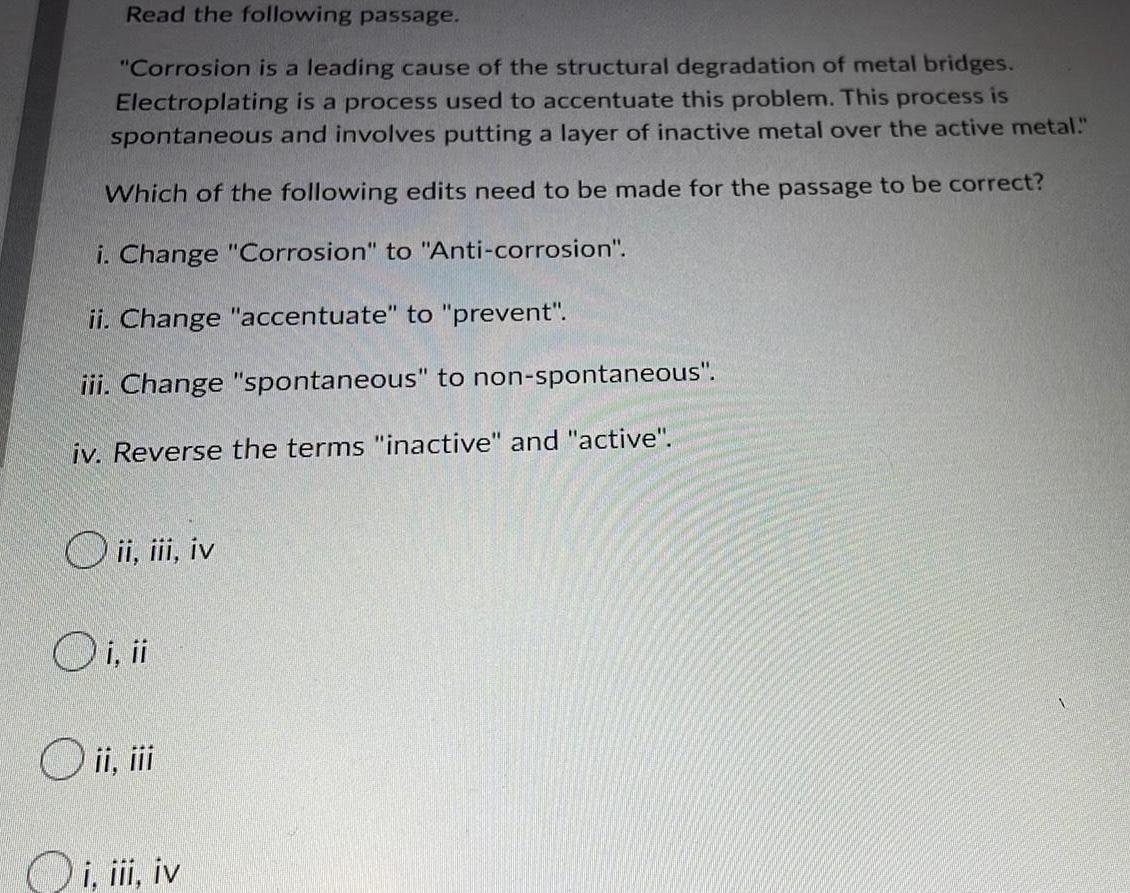Inorganic Chemistry
D Block elements
Read the following passage Corrosion is a leading cause of the structural degradation of metal bridges Electroplating is a process used to accentuate this problem This process is spontaneous and involves putting a layer of inactive metal over the active metal Which of the following edits need to be made for the passage to be correct i Change Corrosion to Anti corrosion ii Change accentuate to prevent iii Change spontaneous to non spontaneous iv Reverse the terms inactive and active O ii iii iv O i ii O ii iii i iii iv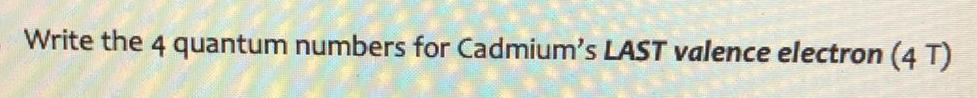Inorganic Chemistry
D Block elements
Write the 4 quantum numbers for Cadmium s LAST valence electron 4 T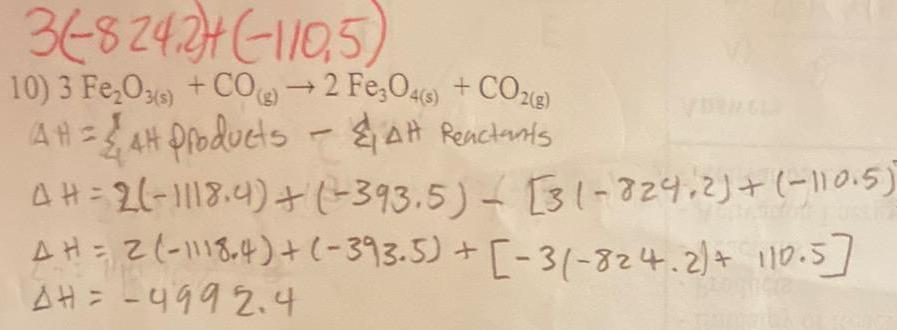Inorganic Chemistry
D Block elements
3 8242 110 5 10 3 Fe O3 s CO g 2 Fe3O4 s CO g AH AH Products AH Reactants 4 H 2 1118 4 393 5 31 824 2 110 5 AH 2 1118 4 393 5 3 824 2 110 5 AH 499 2 4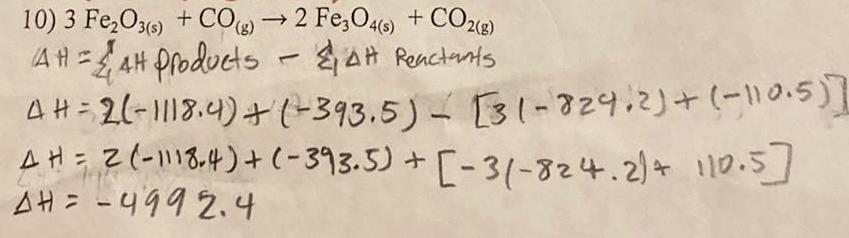Inorganic Chemistry
D Block elements
10 3 Fe O3 s CO g 2 Fe3O4 s CO2 g 4H 4H Products AH Reactants 4H 26 1118 4 393 5 31 829 2 110 5 AH 2 1118 4 393 5 31 824 2 110 5 AH 499 2 4 L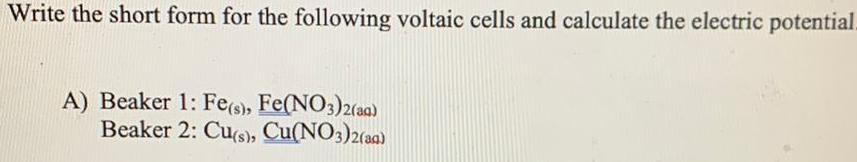Inorganic Chemistry
D Block elements
Write the short form for the following voltaic cells and calculate the electric potential A Beaker 1 Fe s Fe NO3 2 aq Beaker 2 Cu s Cu NO3 2 aq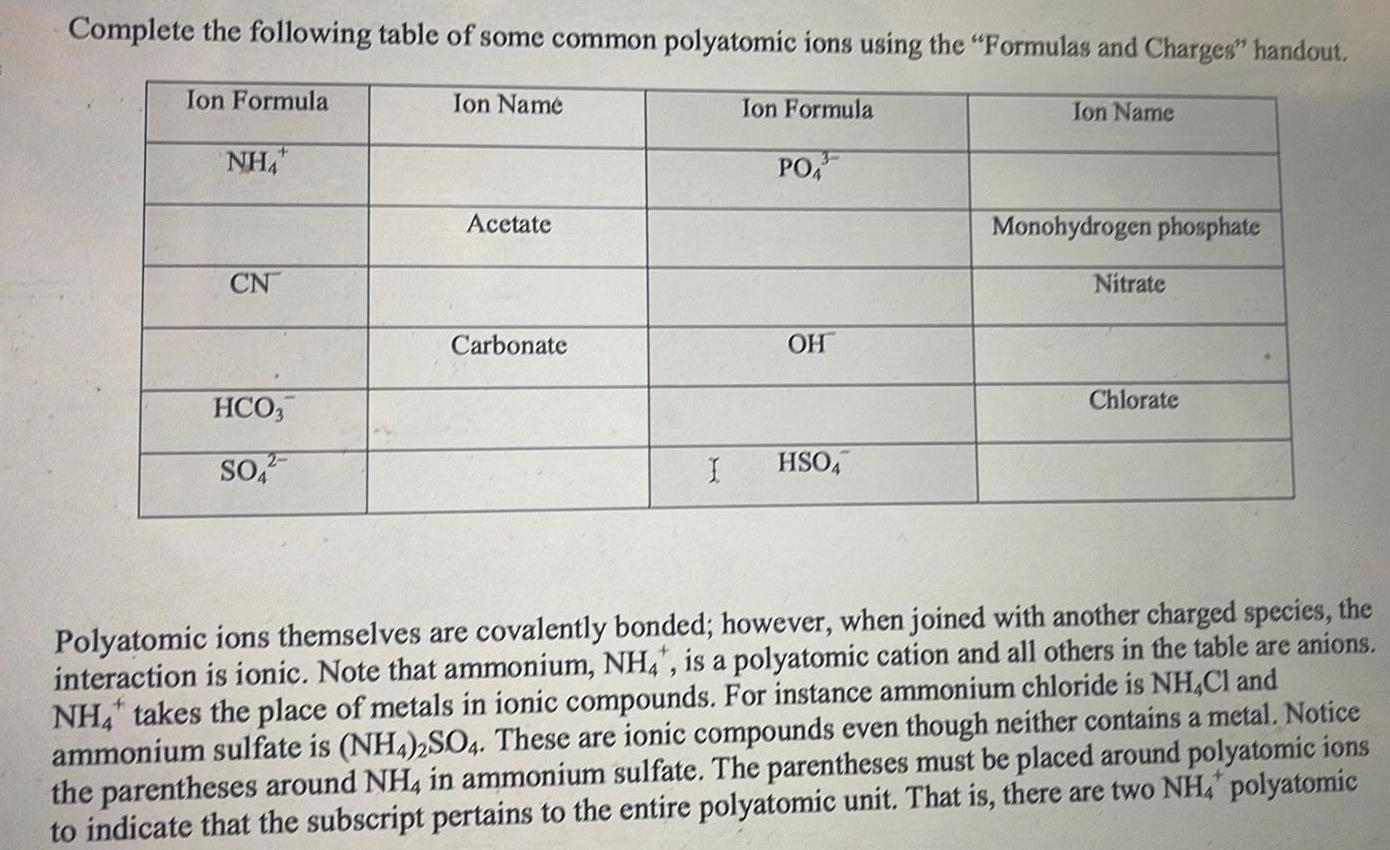Inorganic Chemistry
D Block elements
Complete the following table of some common polyatomic ions using the Formulas and Charges handout Ion Formula NHA CN HCO3 SO Ion Name Acetate Carbonate Ion Formula PO 3 OH I HSO4 Ion Name Monohydrogen phosphate Nitrate Chlorate Polyatomic ions themselves are covalently bonded however when joined with another charged species the interaction is ionic Note that ammonium NH4 is a polyatomic cation and all others in the table are anions NH4 takes the place of metals in ionic compounds For instance ammonium chloride is NH4Cl and ammonium sulfate is NH4 2SO4 These are ionic compounds even though neither contains a metal Notice the parentheses around NH4 in ammonium sulfate The parentheses must be placed around polyatomic ions to indicate that the subscript pertains to the entire polyatomic unit That is there are two NHA polyatomic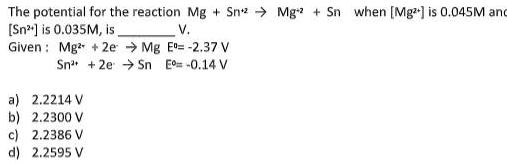Inorganic Chemistry
D Block elements
The potential for the reaction Mg Sn 2 Mg2 Sn when Mg is 0 045M and Sn is 0 035M is V Given Mg 2e Mg E 2 37 V Sn 2e Sn E 0 14 V a 2 2214 V b 2 2300 V c 2 2386 V d 2 2595 V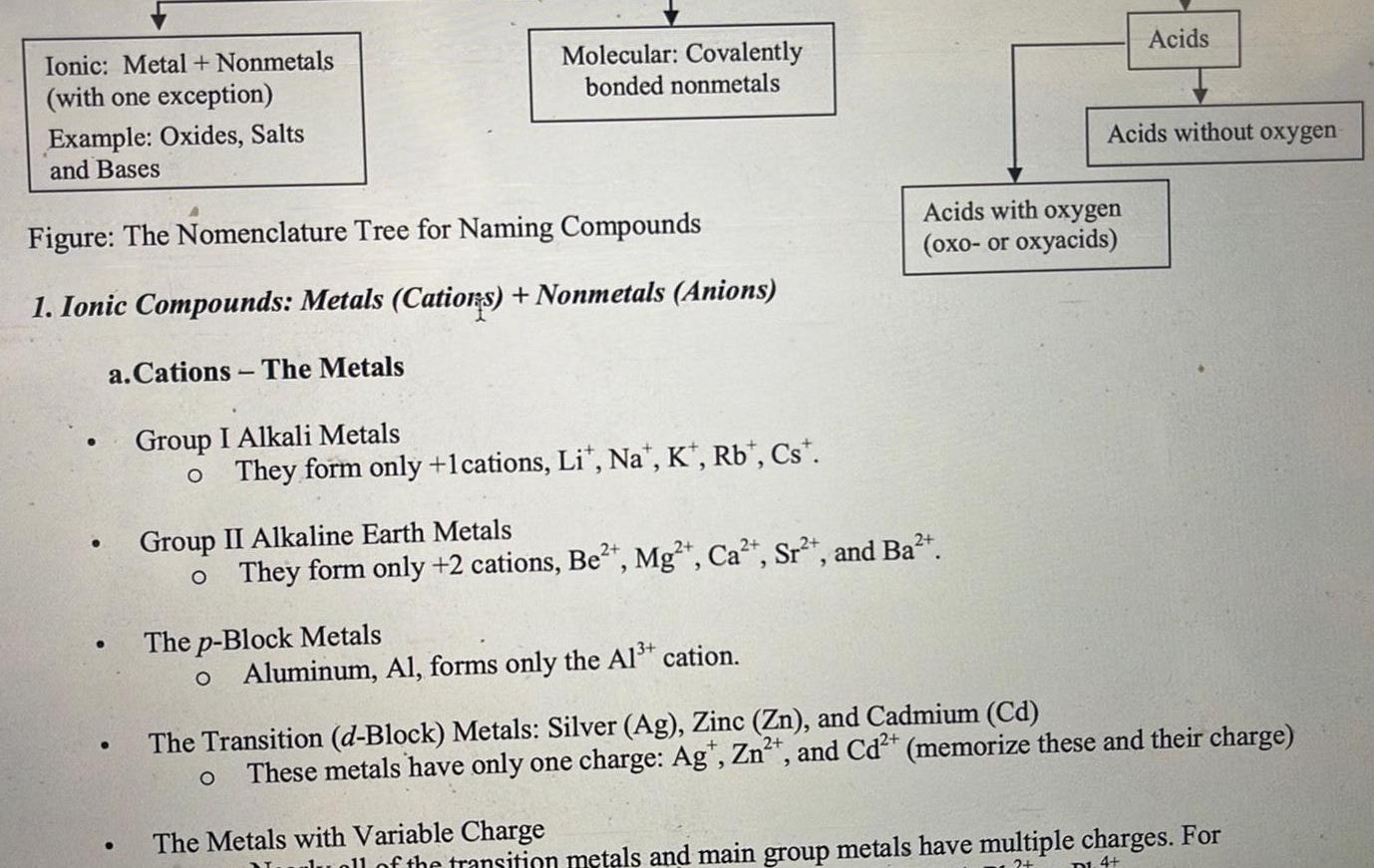Inorganic Chemistry
D Block elements
Ionic Metal Nonmetals with one exception Example Oxides Salts and Bases Figure The Nomenclature Tree for Naming Compounds 1 Ionic Compounds Metals Cations Nonmetals Anions a Cations The Metals Group I Alkali Metals o They form only 1cations Lit Na K Rb Cst Molecular Covalently bonded nonmetals Group II Alkaline Earth Metals o They form only 2 cations Be Mg Ca Sr and Ba The p Block Metals O Aluminum Al forms only the Al cation The Metals with Variable Charge Acids with oxygen oxo or oxyacids Acids Acids without oxygen The Transition d Block Metals Silver Ag Zinc Zn and Cadmium Cd O These metals have only one charge Agt Zn and Cd memorize these and their charge of the transition metals and main group metals have multiple charges For D1 4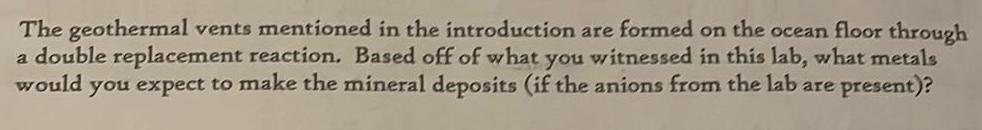Inorganic Chemistry
D Block elements
The geothermal vents mentioned in the introduction are formed on the ocean floor through a double replacement reaction Based off of what you witnessed in this lab what metals would you expect to make the mineral deposits if the anions from the lab are present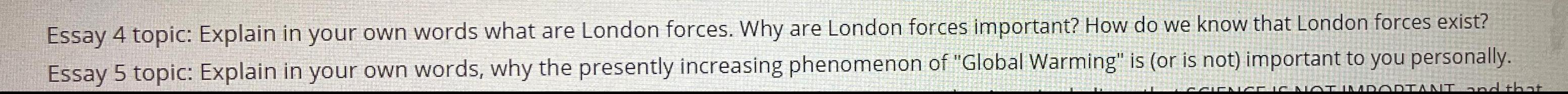Inorganic Chemistry
D Block elements
Essay 4 topic Explain in your own words what are London forces Why are London forces important How do we know that London forces exist Essay 5 topic Explain in your own words why the presently increasing phenomenon of Global Warming is or is not important to you personally CIENCE IS NOT IMPORTANT and that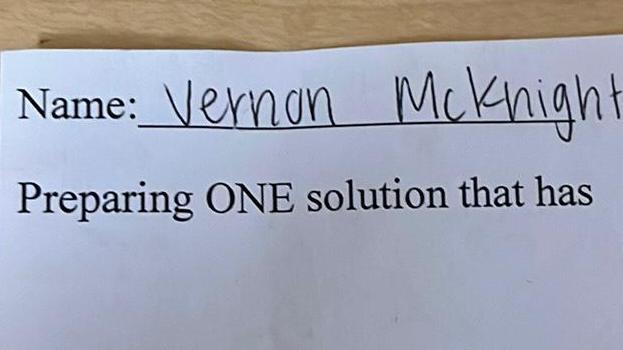Inorganic Chemistry
D Block elements
Name Vernon Mcknight Preparing ONE solution that has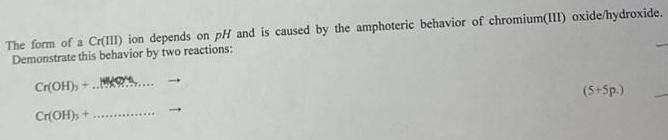Inorganic Chemistry
D Block elements
The form of a Cr III ion depends on pH and is caused by the amphoteric behavior of chromium III oxide hydroxide Demonstrate this behavior by two reactions Cr OH Cr OH 5 5p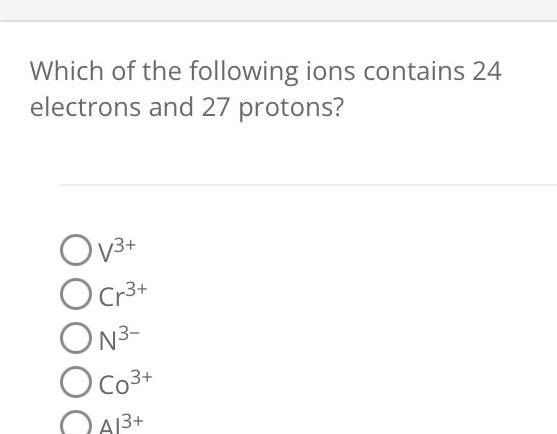Inorganic Chemistry
D Block elements
Which of the following ions contains 24 electrons and 27 protons Ov O Cr ON O CO A1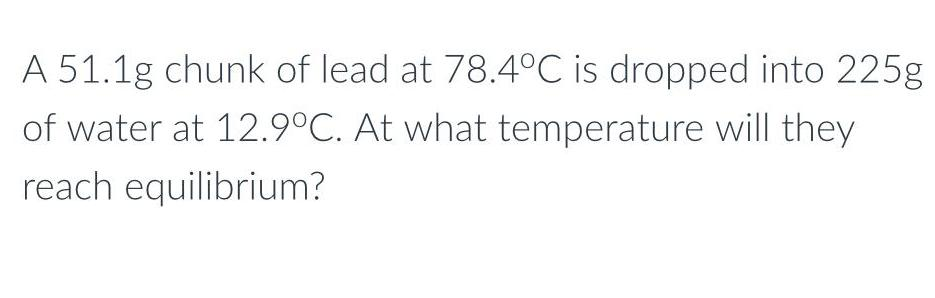Inorganic Chemistry
D Block elements
A 51 1g chunk of lead at 78 4 C is dropped into 225g of water at 12 9 C At what temperature will they reach equilibrium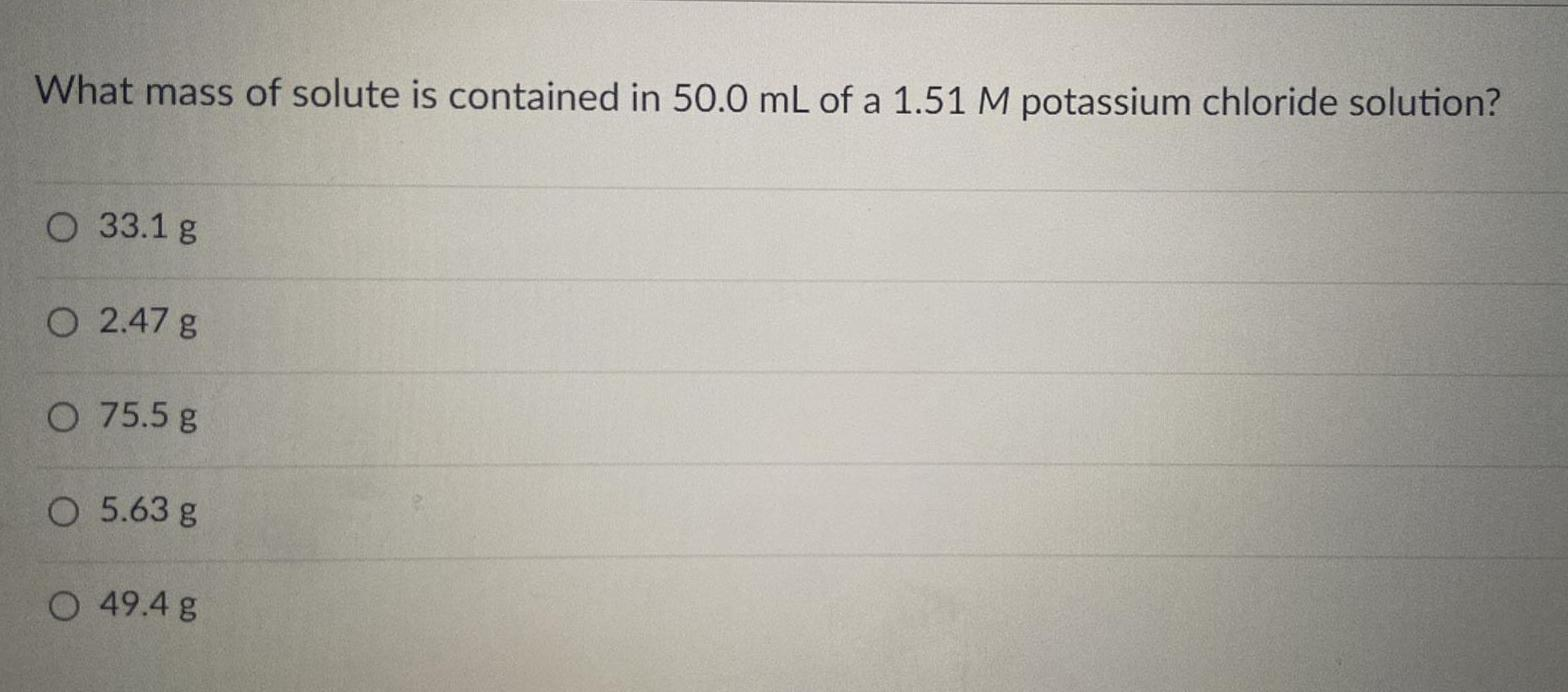Inorganic Chemistry
D Block elements
What mass of solute is contained in 50 0 mL of a 1 51 M potassium chloride solution O 33 1 g O 2 47 g O 75 5 g O 5 63 g O 49 4 g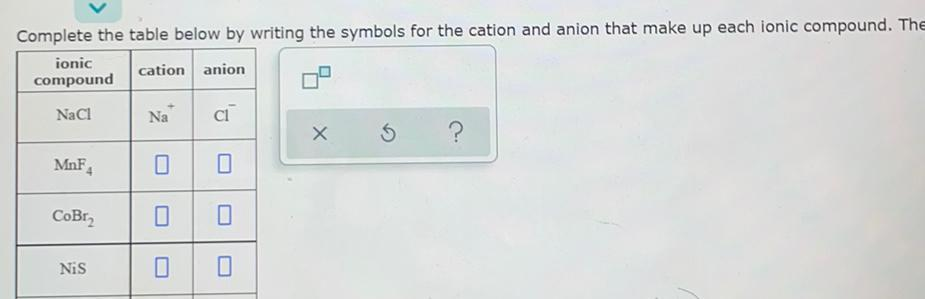Inorganic Chemistry
D Block elements
Complete the table below by writing the symbols for the cation and anion that make up each ionic compound The ionic cation anion compound NaCl MnF4 CoBr Nis Na 0 CI 0 X S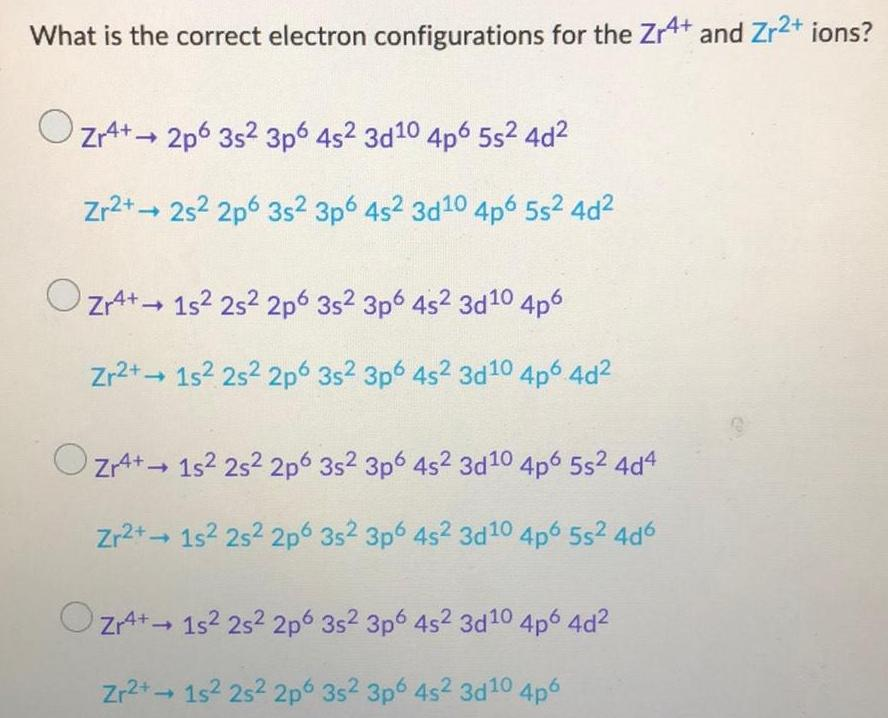Inorganic Chemistry
D Block elements
What is the correct electron configurations for the Zr4+ and Zr²+ ions? Zr4+2p6 35² 3p6 4s² 3d10 4p6 5s² 4d² Zr2+2s² 2p 3s² 3p6 4s2 3d10 4p6 5s² 4d² Zr4+15² 25² 2p 3s² 3p6 4s² 3d10 4p6 Zr2+ 1s² 2s2 2p 3s² 3p6 4s² 3d10 4p6 4d² Zr4+ 15² 25² 2p6 35² 3p6 4s² 3d10 4p6 5s² 4d4 Zr2+1s² 2s² 2p 3s2 3p6 4s2 3d10 4p6 5s²4d6 Zr4+ 1s² 2s² 2p Zr2+→ 1s² 25² 2p 3s² 3p6 4s² 3d¹0 4p6 4d² 3s2 3p6 4s² 3d10 4p6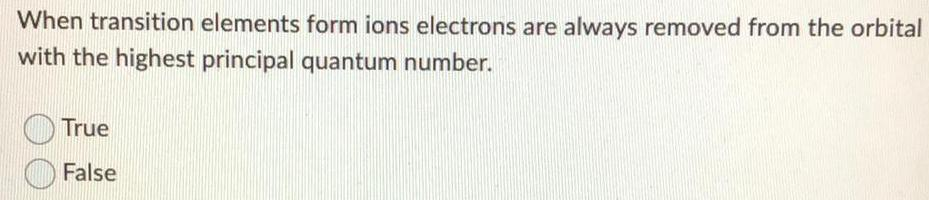Inorganic Chemistry
D Block elements
When transition elements form ions electrons are always removed from the orbital with the highest principal quantum number. True False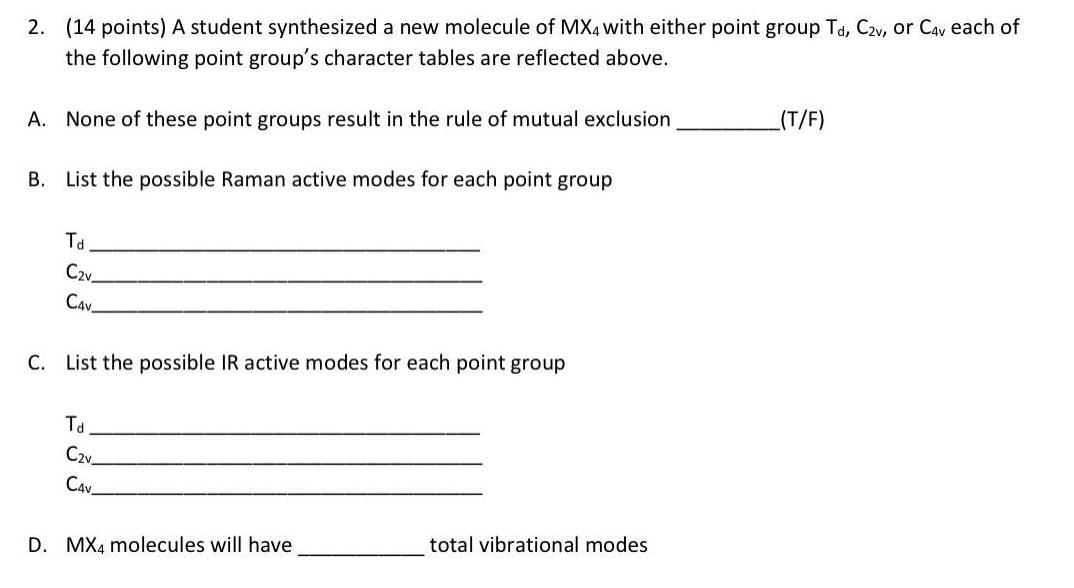Inorganic Chemistry
D Block elements
A student synthesized a new molecule of MX4 with either point group Td, C2v, or C4v each of the following point group's character tables are reflected above. A. None of these point groups result in the rule of mutual exclusion B. List the possible Raman active modes for each point group Td C2v CAV C. List the possible IR active modes for each point group Td C2v C4V D. MX4 molecules will have ____ total vibrational modes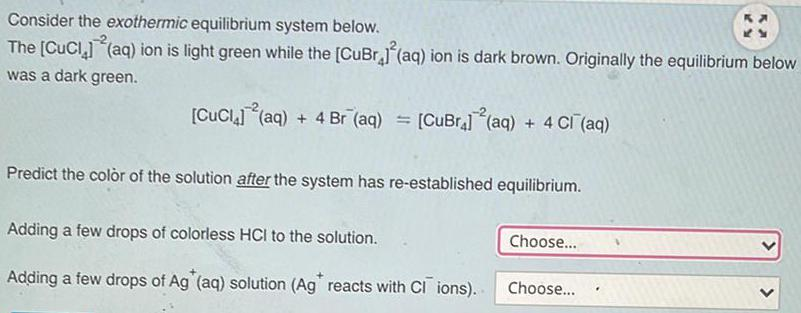Inorganic Chemistry
D Block elements
Consider the exothermic equilibrium system below. The [CuCl4]-2(aq) ion is light green while the [CuBr4]2 (aq) ion is dark brown. Originally the equilibrium below was a dark green. [CuCl4]-2(aq) + 4 Br-(aq) = [CuBr4}-2(aq) + 4 Cl-(aq) Predict the color of the solution after the system has re-established equilibrium. Adding a few drops of colorless HCI to the solution. Adding a few drops of Ag (aq) solution (Ag reacts with CI ions).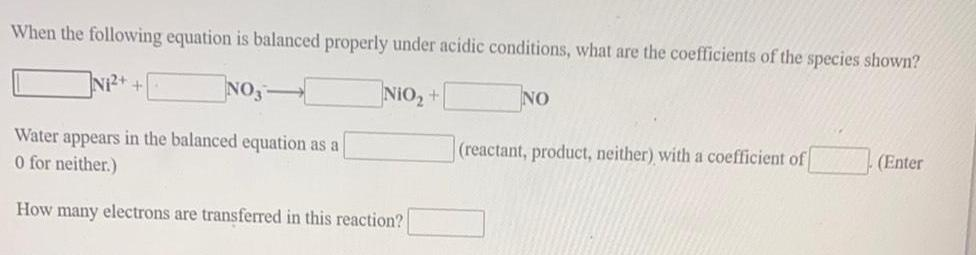Inorganic Chemistry
D Block elements
When the following equation is balanced properly under acidic conditions, what are the coefficients of the species shown? N₁²+ + NO3- NIO₂ + NO Water appears in the balanced equation as a 0 (reactant,product,neither) with a coefficient of (enter 0 or neither) How many electrons are transferred in this reaction?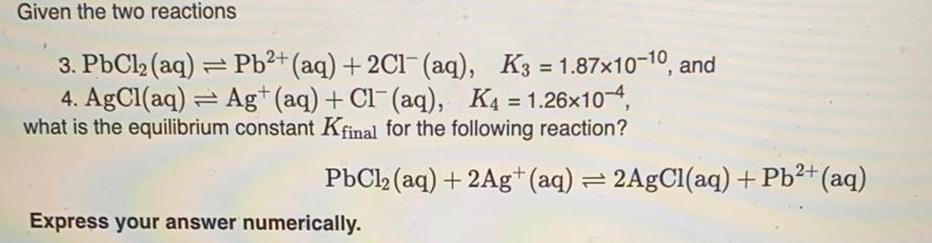Inorganic Chemistry
D Block elements
Given the two reactions 3. PbCl₂ (aq) = Pb²+ (aq) + 2Cl¯ (aq), K3=1.87x10-10, and 4. AgCl(aq) Ag+ (aq) + Cl(aq), K4 = 1.26x10-4, what is the equilibrium constant Kfinal for the following reaction? PbCl₂ (aq) + 2Ag+ (aq) = 2AgCl(aq) + Pb²+ (aq) Express your answer numerically.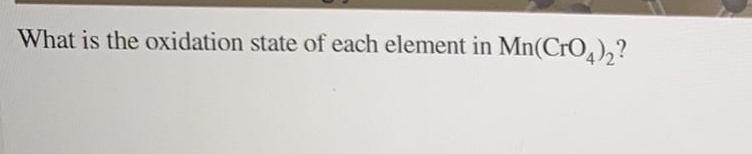Inorganic Chemistry
D Block elements
What is the oxidation state of each element in Mn(CrO4)₂?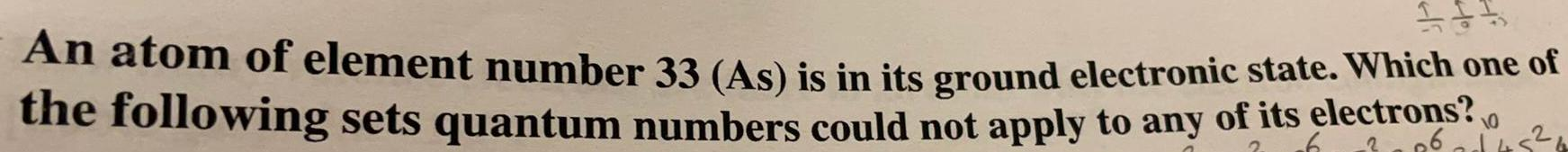Inorganic Chemistry
D Block elements
An atom of element number 33 (As) is in its ground electronic state. Which one of the following sets quantum numbers could not apply to any of its electrons?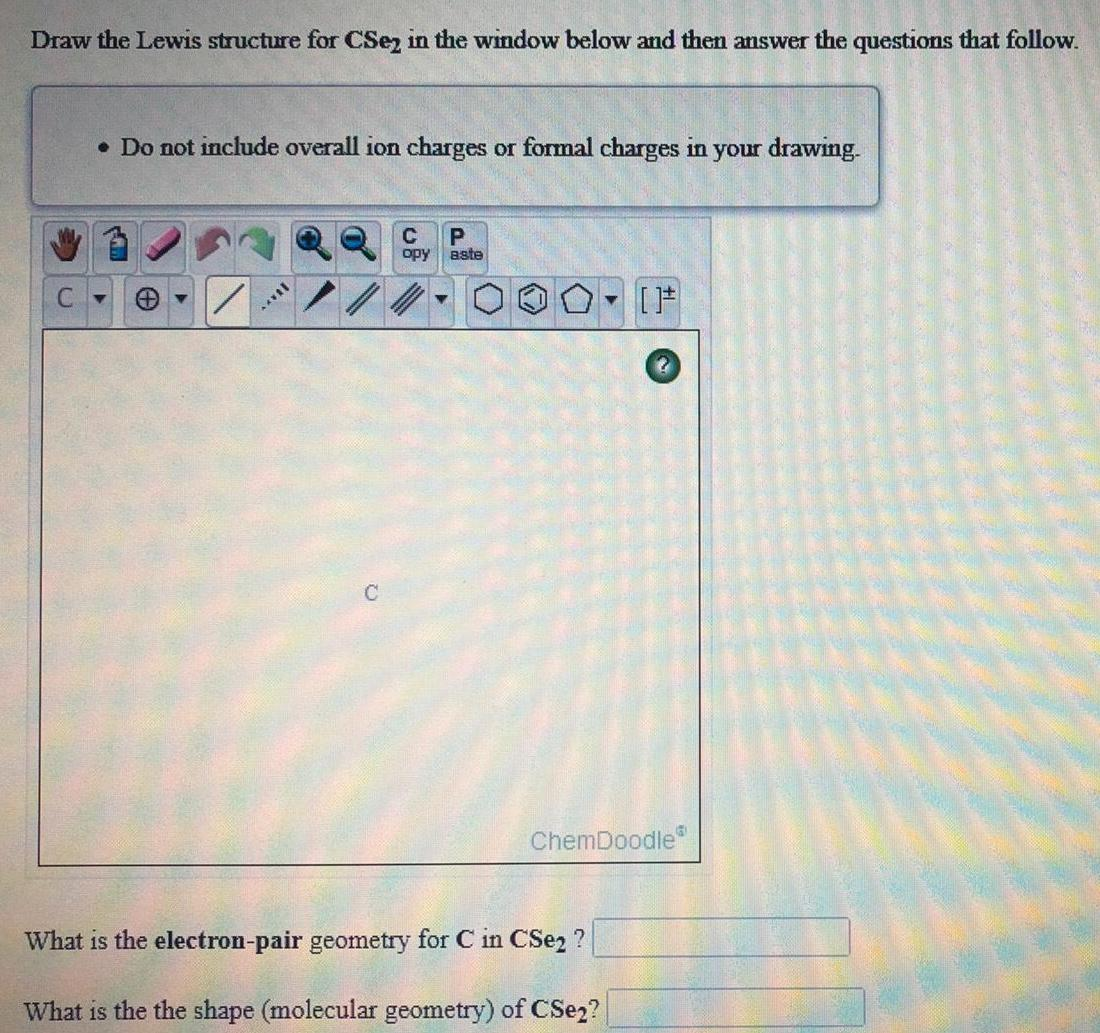Inorganic Chemistry
D Block elements
Draw the Lewis structure for CSe2 in the window below and then answer the questions that follow.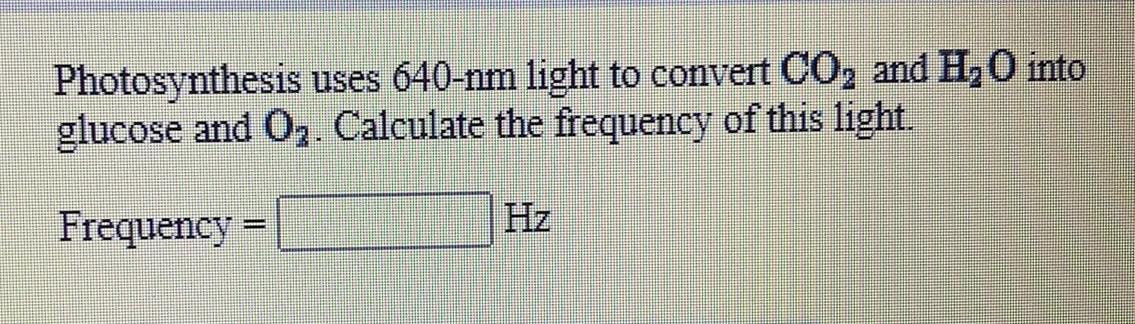Inorganic Chemistry
D Block elements
Photosynthesis uses 640-nm light to convert CO₂ and H₂O into glucose and O₂. Calculate the frequency of this light.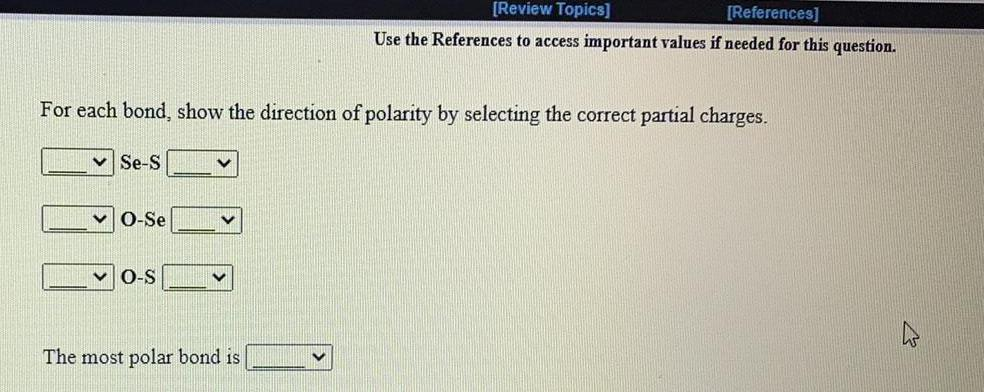Inorganic Chemistry
D Block elements
For each bond, show the direction of polarity by selecting the correct partial charges. Se-S O-Se O-S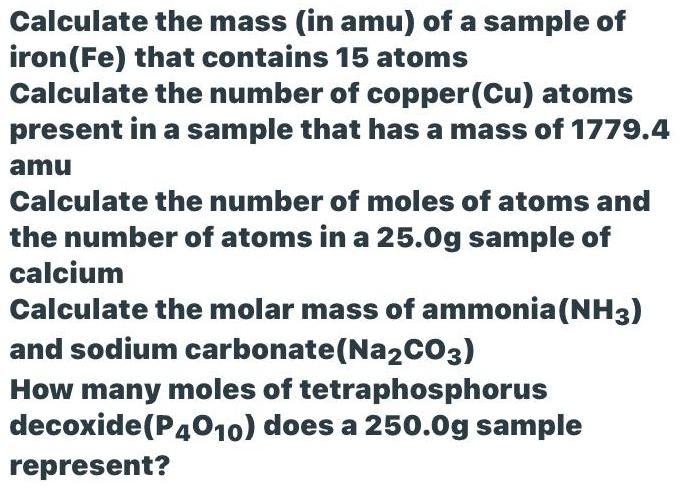Inorganic Chemistry
D Block elements
Calculate the mass (in amu) of a sample of iron (Fe) that contains 15 atoms Calculate the number of copper (Cu) atoms present in a sample that has a mass of 1779.4 amu Calculate the number of moles of atoms and the number of atoms in a 25.0g sample of calcium Calculate the molar mass of ammonia (NH3) and sodium carbonate (Na2CO3) How many moles of tetraphosphorus decoxide (P4010) does a 250.0g sample represent?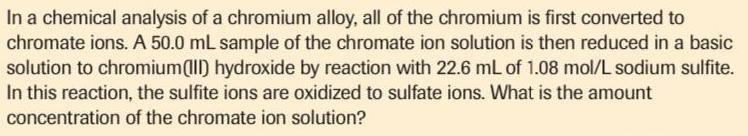Inorganic Chemistry
D Block elements
In a chemical analysis of a chromium alloy, all of the chromium is first converted to chromate ions. A 50.0 mL sample of the chromate ion solution is then reduced in a basic solution to chromium(III) hydroxide by reaction with 22.6 mL of 1.08 mol/L sodium sulfite. In this reaction, the sulfite ions are oxidized to sulfate ions. What is the amount concentration of the chromate ion solution?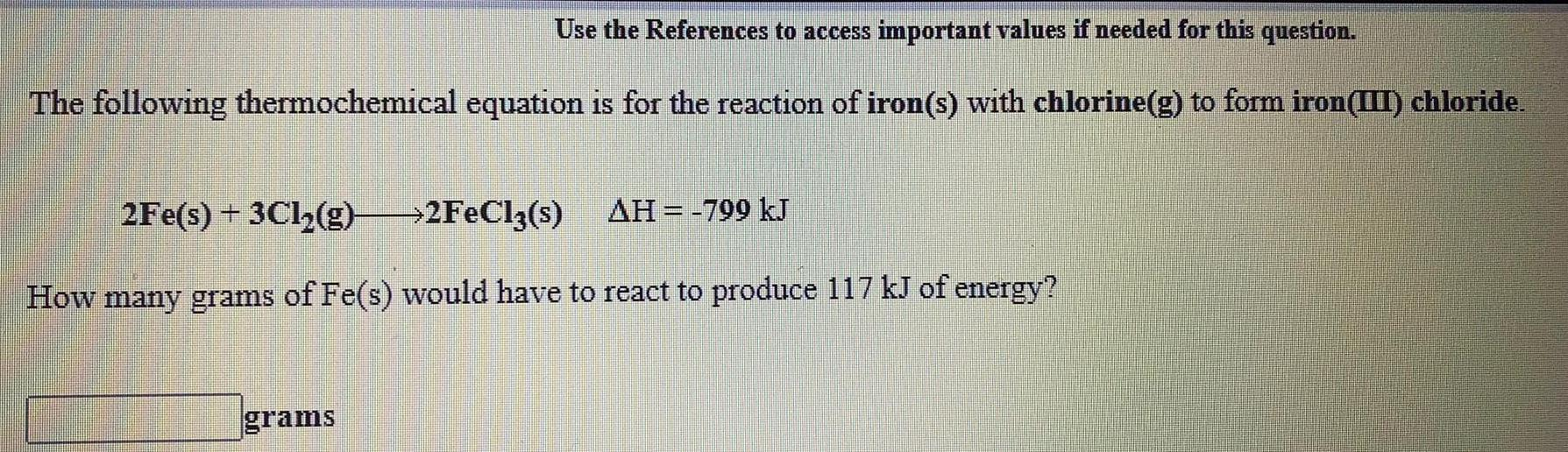Inorganic Chemistry
D Block elements
The following thermochemical equation is for the reaction of iron(s) with chlorine(g) to form iron(III) chloride. 2Fe(s) + 3Cl₂(g) → 2FeCl3(s) ΔH = -799 kJ How many grams of Fe(s) would have to react to produce 117 kJ of energy?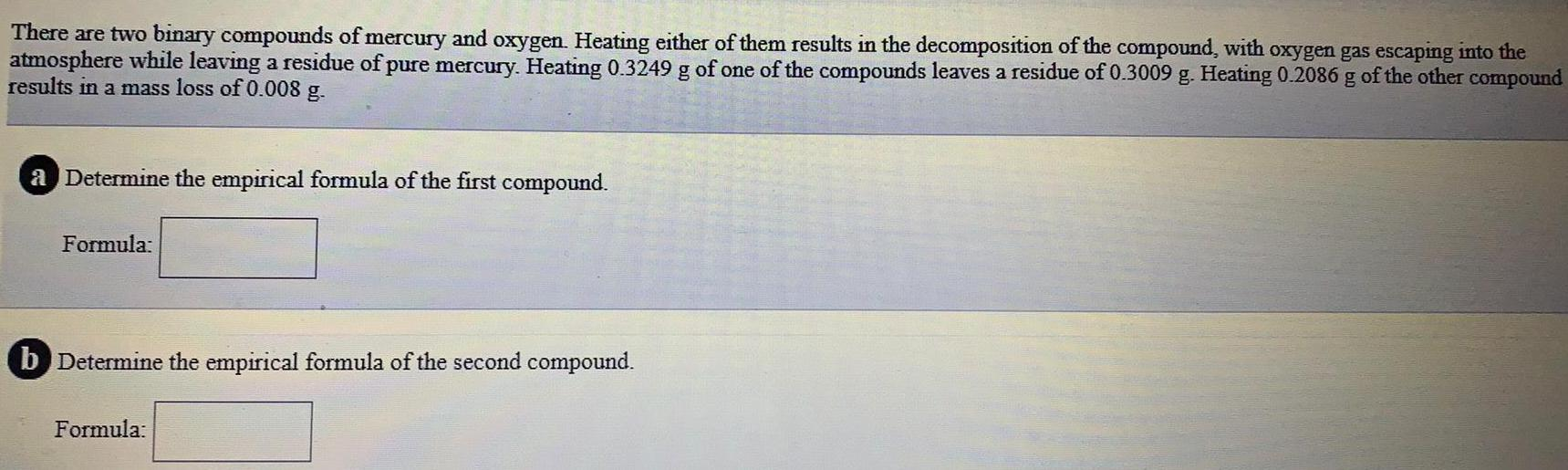Inorganic Chemistry
D Block elements
There are two binary compounds of mercury and oxygen. Heating either of them results in the decomposition of the compound, with oxygen gas escaping into the atmosphere while leaving a residue of pure mercury. Heating 0.3249 g of one of the compounds leaves a residue of 0.3009 g. Heating 0.2086 g of the other compound results in a mass loss of 0.008 g. a Determine the empirical formula of the first compound. Formula: b Determine the empirical formula of the second compound. Formula: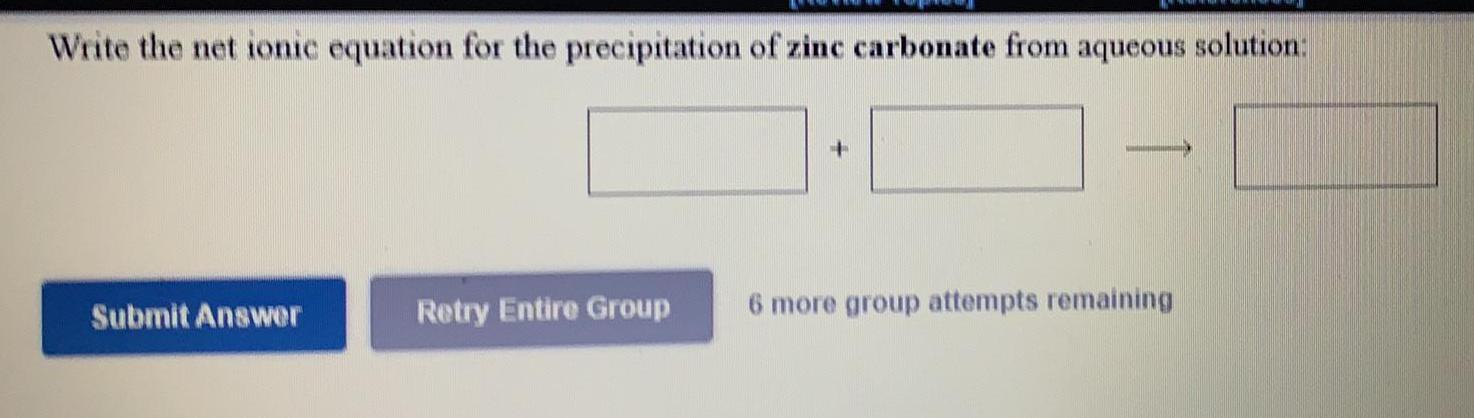Inorganic Chemistry
D Block elements
Write the net ionic equation for the precipitation of zinc carbonate from aqueous solution: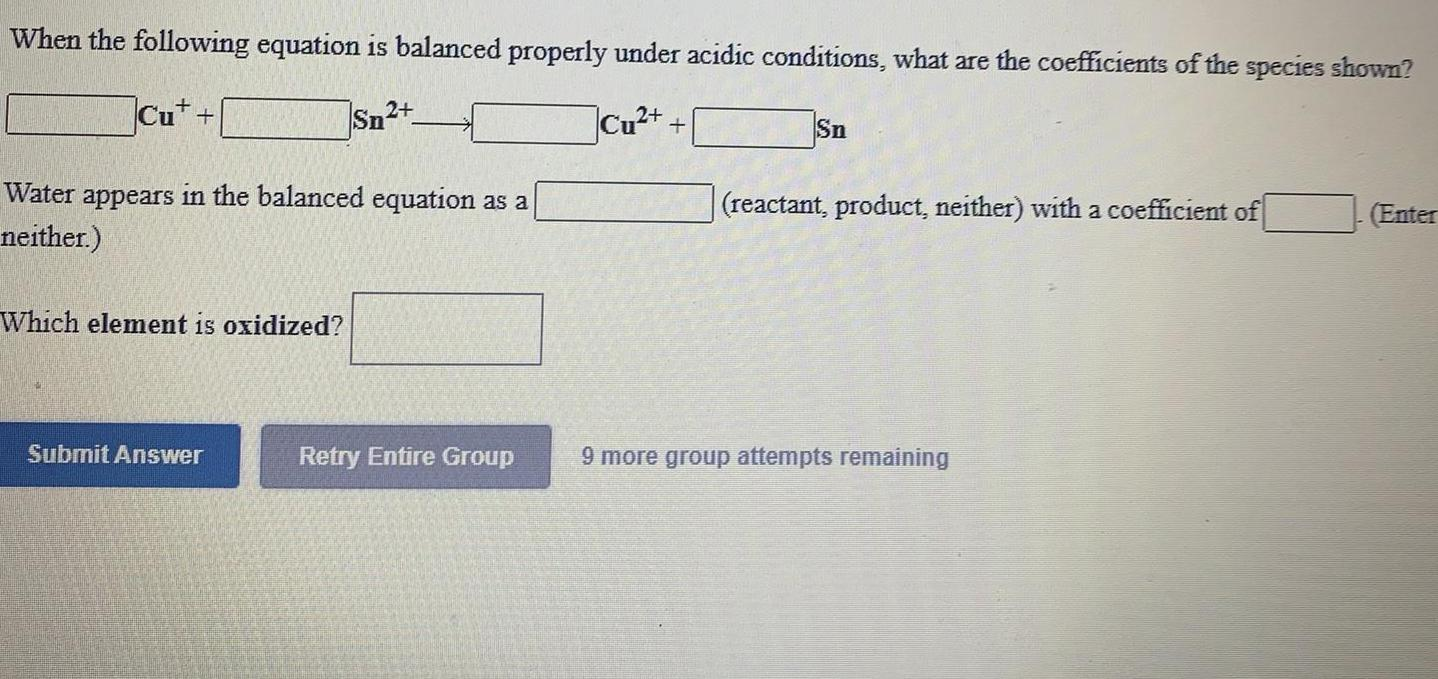Inorganic Chemistry
D Block elements
When the following equation is balanced properly under acidic conditions, what are the coefficients of the species shown? Cu+ + Sn2+ -> __ Cu2+ + __Sn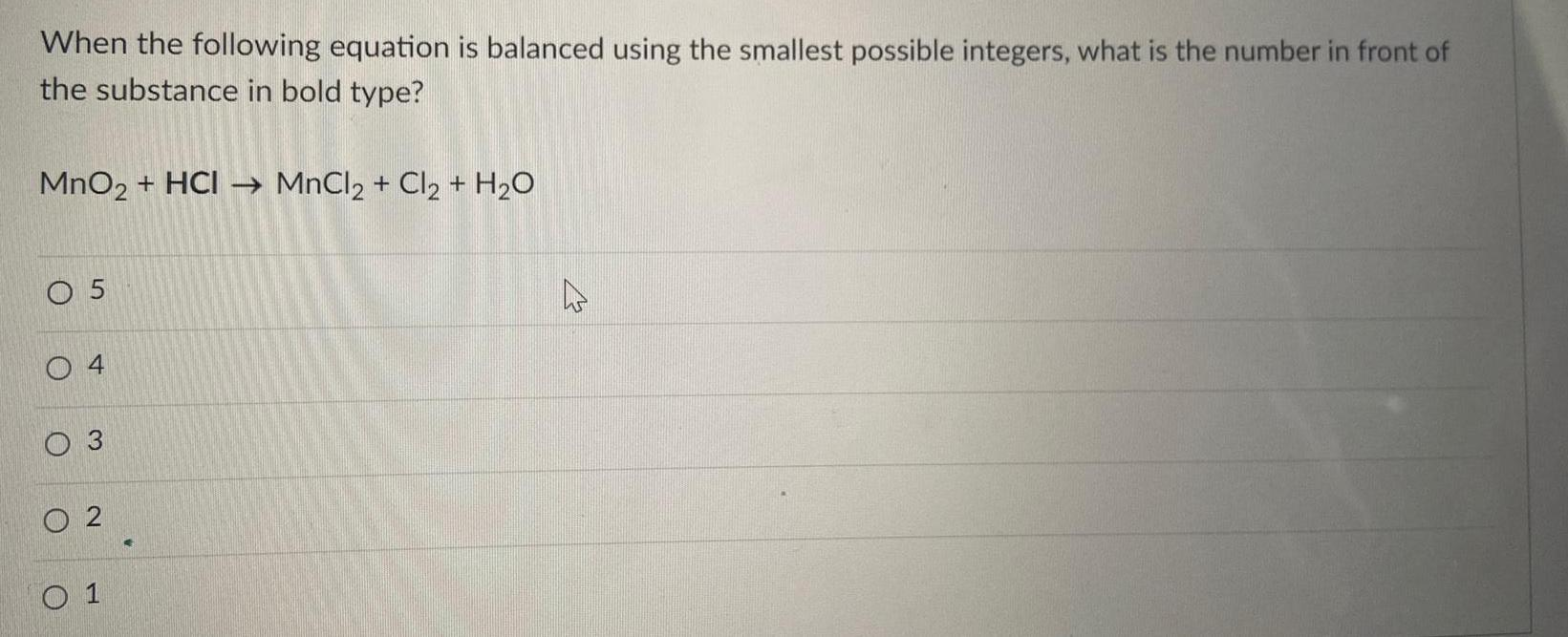Inorganic Chemistry
D Block elements
When the following equation is balanced using the smallest possible integers, what is the number in front of the substance in bold type? MnO2 + HCI →→MnCl2 + Cl2 + H₂O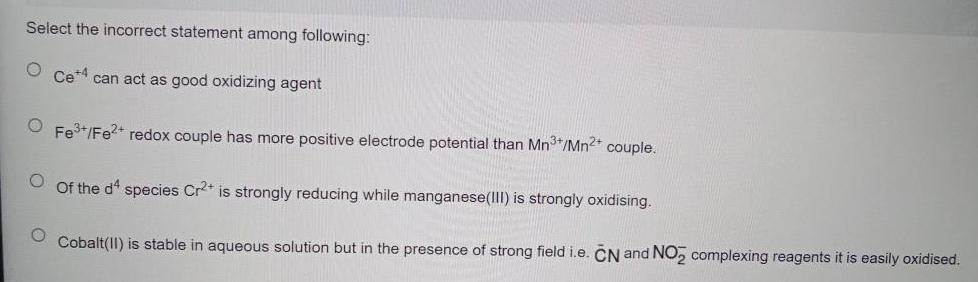Inorganic Chemistry
D Block elements
Select the incorrect statement among following: Ce4+ can act as good oxidizing agent Fe3+ / Fe2+ redox couple has more positive electrode potential than Mn3+/Mn2+ couple. Of the d4 species Cr2+ is strongly reducing while manganese(III) is strongly oxidising. Cobalt(II) is stable in aqueous solution but in the presence of strong field i.e. CN and NO2- complexing reagents it is easily oxidised.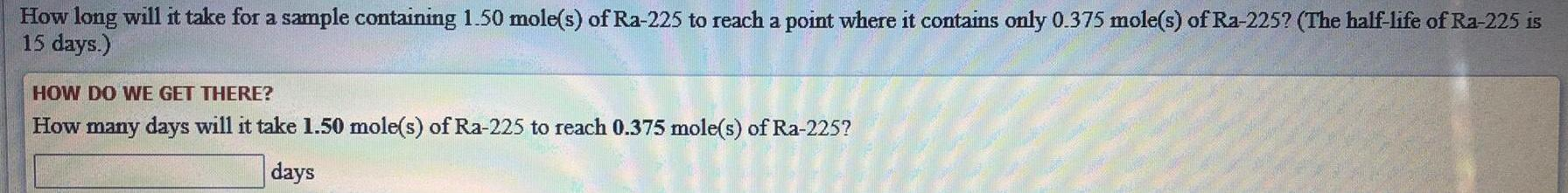Inorganic Chemistry
D Block elements
How long will it take for a sample containing 1.50 mole(s) of Ra-225 to reach a point where it contains only 0.375 mole(s) of Ra-225? (The half-life of Ra-225 is15 days.
1